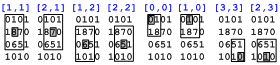Home Contents << Prev >> Next## Image Processing 1: Filters

1.3    The Fog Filter
1.3.1 The 3x3 Fog Filter
1.3.2 Fog Filtering Of Real Images
1.3.3 Questions & Answers
Copyright © by V. Miszalok, last update: 2010-11-05Special Effect: FogLet me know
what you think

 You can download the complete source codes of the Silverlight solution directories of this chapter. When you have installed Visual Web Developer 2010 Express plus Web Platform, you unzip the directories and click ***.sln.

## The 3x3 Fog Filter

is a variant of the 3x3 avarage filter, which is too strong for many applications.
The fog filter adds a certain percentage of the original pixel to the democratic vote of its 3x3 rectangular neighborhood.
Thus the central pixel obtains a higher vote than any of its 8 neighbors.
Here is the pseudo-code for a gray value pixel:
1. Take the pixel's gray value and add the gray values of its eight neighbours.
2. Divide the sum by 9, round the result to integer.
3. Write it to an intermediary image.
4. The output image is composed of a certain FilterWeight-percentage of the intermediary image plus
a certain OriginalWeight-percentage of the input image.

The addition of a percentage of the original image is equivalent to an elevated weight of the pixel[y,x] among its neighbors.
Sample: The 3x3 fog filter which gives old[y,x] a wight of 8, what means, that old[y,x] has as many votes as its 8 neighbors together
→ equivalent to 50% original + 50% average filter.

 ``` 0101 old = 1870 0651 1010``````new[1,1] = (0 + 1 + 0 + 1 + 8*8 + 7 + 0 + 6 + 5)/16 = 84/16 = 5.25 = rounded 5 new[2,1] = (1 + 0 + 1 + 8 + 7*8 + 0 + 6 + 5 + 1)/16 = 77/16 = 4.81 = rounded 5 new[1,2] = (1 + 8 + 7 + 0 + 6*8 + 5 + 1 + 0 + 1)/16 = 69/16 = 4.31 = rounded 4 new[2,2] = (8 + 7 + 0 + 6 + 5*8 + 1 + 0 + 1 + 0)/16 = 60/16 = 3.75 = rounded 4 new[0,0] = ( 0*8 + 1 + 1 + 8)/11 = 10/11 = 0.91 = rounded 1 new[1,0] = ( 0 + 1*8 + 0 + 1 + 8 + 7)/13 = 24/13 = 1.85 = rounded 2 new[3,3] = (5 + 1 + 1 + 0*8 )/11 = 7/11 = 0.63 = rounded 1 new[2,3] = (6 + 5 + 1 + 0 + 1*8 + 0 )/13 = 20/13 = 1.54 = rounded 2 etc.``` ``` 1331 new = 2551 1442 1121``` The gray value differences of the original remain visible, and image "new" is less blurred as with the 3x3 average filter.

******************************************************************************************************
Experiment: After drawing, drag the mixture slider between 100% filter and 100% original.
******************************************************************************************************

```//C#-Code of the FogFilter:
Byte[,] image0 = new Byte[ySize,xSize]; // global input image
Byte[,] image1 = new Byte[ySize,xSize]; // contains the result of the AverageFilter3x3
Byte[,] image2 = new Byte[ySize,xSize]; // global output image
double FilterWeight   = AverageFilterPrevalenceInPercent / 100.0;
double OriginalWeight = 1.0 - FilterWeight;
for ( int y=1; y < ySize-1; y++ )
for ( int x=1; x < xSize-1; x++ )
image2[y,x] = Convert.ToByte( image1[y,x]*FilterWeight +
image0[y,x]*OriginalWeight );
DrawImage( image2 );  ```

## Fog Filtering Of Real Images

******************************************************************************************************
Experiments:
1. Drag the mixture slider between 100% original and 0% original.
2. Drag the mixture slider to 100% and notice that the FWidth- and FHeight-sliders don't change anything.
3. Drag both the FWidth- and FHeight-sliders to 1 and notice that the mixture slider doesn't change anything.
4. A good mysty atmosphere is obtained with a big kernel and a low original.
******************************************************************************************************

## Questions & Answers

******************************************************************************************************
Q: What is a Fog Filter ? Benefit ?
A: A normal average filter with an additional feature: pixel[y,x] counts more than any of its neighbors.
It softens and fine tunes the brutal averaging filter.
******************************************************************************************************
Q: What means "Fog" ?
A: Fog filters mimic the effect of natural fog, creating a soft glow and flare.
They produce a warm romantic tone or a mysty atmosphere.
******************************************************************************************************
Q: Pseudo code of 3x3 fog filtering of an image0 to image1 to image2
with FilterWeight+OriginalWeight == 1.0 ?

```A: for any row y
for any column x
{ for any yy=-1 to yy=1
for any xx=-1 to xx=1
sum += image0[y+yy,x+xx];
image1[y,x] = sum / 9;
image2[y,x] = FilterWeight*image1[y,x] + OriginalWeight*image0[y,x];
}```

******************************************************************************************************
Q: Pseudo code of 21x21 fog filtering of image0 to image1 to image2
with FilterWeight+OriginalWeight == 1.0 ?

```A: for any row y
for any column x
{ for any yy=-10 to yy=10
for any xx=-10 to xx=10
sum += image0[y+yy,x+xx];
image1[y,x] = sum / (21*21);
image2[y,x] = FilterWeight*image1[y,x] + OriginalWeight*image0[y,x];
}```

 Home Contents << Prev >> Next